edHelper.com Math Critical Thinking Geometry Drawing Shapes Challenge Lesson - Dot Game - Printable Activity Lesson Plan and Worksheets Two Player Drawing Shapes Challenge Lesson ActivityWorksheets, Lessons, and Printables

Drawing Shapes Challenge - Dot to Dot Game: Two-Player Activity Game
Students take turn drawing lines to make shapes

Drawing One Shape - Rectangles, Squares, and Triangles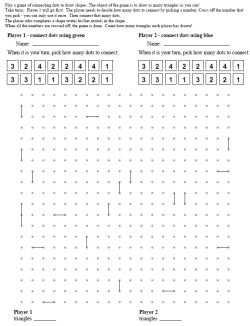Easiest: Drawing One Shape Two Player Game (Rectangles, Squares, and Triangles) - Some Lines Given Easiest: Drawing One Shape Two Player Game (Rectangles, Squares, and Triangles) - No Lines Given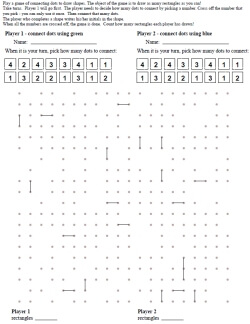Drawing One Shape Two Player Game (Rectangles, Squares, and Triangles) - Some Lines Given - With Missing Dots (25% missing) Drawing One Shape Two Player Game (Rectangles, Squares, and Triangles) - Some Lines Given - With Missing Dots (50% missing)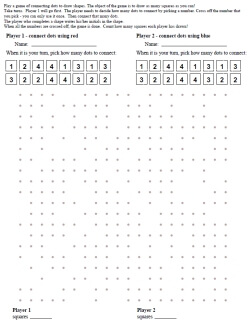Drawing One Shape Two Player Game (Rectangles, Squares, and Triangles) - No Lines Given - With Missing Dots (25% missing) Drawing One Shape Two Player Game (Rectangles, Squares, and Triangles) - No Lines Given - With Missing Dots (50% missing)

Drawing One Shape - Any ShapeEasiest: Drawing One Shape Two Player Game (Any Shape) - Some Lines Given Easiest: Drawing One Shape Two Player Game (Any Shape) - No Lines GivenDrawing One Shape Two Player Game (Any Shape) - Some Lines Given - With Missing Dots (25% missing) Drawing One Shape Two Player Game (Any Shape) - Some Lines Given - With Missing Dots (50% missing)Drawing One Shape Two Player Game (Any Shape) - No Lines Given - With Missing Dots (25% missing) Drawing One Shape Two Player Game (Any Shape) - No Lines Given - With Missing Dots (50% missing)

Drawing Two Shapes - Any Shape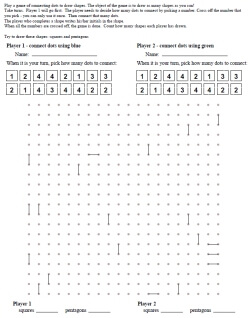Drawing Two Shapes Two Player Game (Any Shape) - Some Lines Given Drawing Two Shapes Two Player Game (Any Shape) - No Lines Given Drawing Two Shapes Two Player Game (Any Shape) - Some Lines Given - Points Given to Shapes Drawing Two Shapes Two Player Game (Any Shape) - No Lines Given - Points Given to Shapes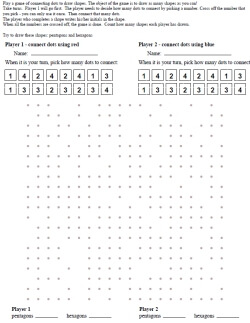Drawing Two Shapes Two Player Game (Any Shape) - No Lines Given - With Missing Dots (25% missing) Drawing Two Shapes Two Player Game (Any Shape) - No Lines Given - With Missing Dots (50% missing) Drawing Two Shapes Two Player Game (Any Shape) - No Lines Given - With Missing Dots (25% missing) - Points Given to Shapes Drawing Two Shapes Two Player Game (Any Shape) - No Lines Given - With Missing Dots (50% missing) - Points Given to Shapes

Drawing Four Shapes - Any Shape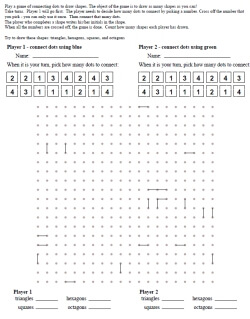Drawing Four Shapes Two Player Game (Any Shape) - Some Lines Given Drawing Four Shapes Two Player Game (Any Shape) - No Lines Given Drawing Four Shapes Two Player Game (Any Shape) - Some Lines Given - Points Given to Shapes Drawing Four Shapes Two Player Game (Any Shape) - No Lines Given - Points Given to Shapes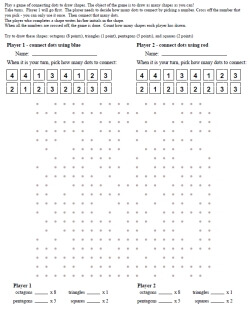Drawing Four Shapes Two Player Game (Any Shape) - No Lines Given - With Missing Dots (25% missing) Drawing Four Shapes Two Player Game (Any Shape) - No Lines Given - With Missing Dots (50% missing) Drawing Four Shapes Two Player Game (Any Shape) - No Lines Given - With Missing Dots (25% missing) - Points Given to Shapes Drawing Four Shapes Two Player Game (Any Shape) - No Lines Given - With Missing Dots (50% missing) - Points Given to Shapes

Drawing Shapes Challenge

Have a suggestion or would like to leave feedback?